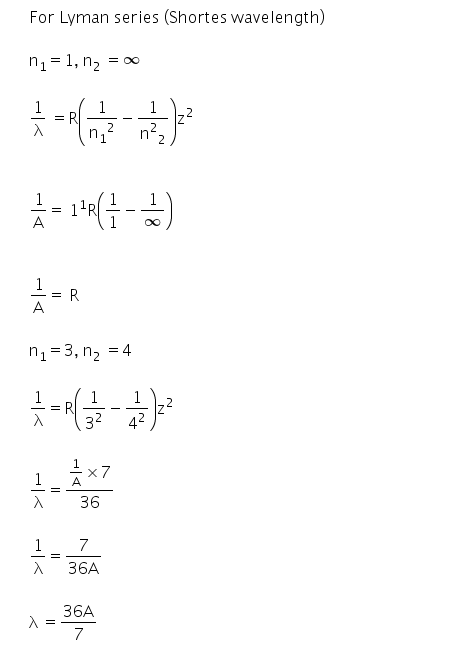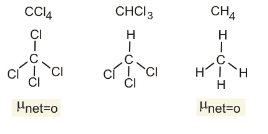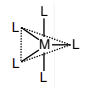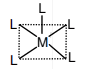Filter By

## All Questions

#### The group having triangular planar structures is :   Option: 1Option: 2Option: 3Option: 4The group having triangular planar structures is CO32-, NO?????3?-,SO?3
In CO32- ion, C atom is sp?????2 hybridised. This results in triangular planar structure.
In NO?????3????- ion, N atom is sp?????2 hybridised. This results in triangular planar structure.
In SO?????3, S atom is sp?????2 hybridised. This results in triangular planar structure.
In all above molecules/ions, the central atom has 3 bonding domains and bond angle of 120o.

#### The electron in the hydrogen atom undergoes transition from higher orbitals to orbital of radius 211.6 pm.  This transition is associated with : Option: 1  Lyman series Option: 2  Balmer series Option: 3  Paschen series Option: 4  Brackett series

For hydrogen atom, the radius of n??????th orbit, r??????n = 52.9 pm ×n2

211.6 pm = 52.9 pm×n2

n??????2=4

n=2

Hence, the transition is from a higher orbit to the second orbit.
This corresponds to the Balmer series.

## Crack CUET with india's "Best Teachers"

• HD Video Lectures
• Unlimited Mock Tests
• Faculty Support#### If the shortest wavelength in Lyman series of hydrogen atom is A, then the longest wavelength in Paschen series of He+ is :   Option: 1 Option: 2 Option: 3  Option: 4

we know that,#### For the Balmer series in the spectrum of H atom, the correct statements among (I) to (IV) are: (1) As wavelength decreases, the lines in the series converge (II) The integer n1 is equal to 2 (III) The lines of longest wavelength corresponds to n2 = 3 (IV) The ionization energy of hydrogen can be calculated from wavenumber of these lines   Option: 1Option: 2Option: 3Option: 4Line Spectrum of Hydrogen-like atomsWhere R is called Rydberg constant, R = 1.097 X 107,  Z is the atomic number

n1= 1, 2, 3….

n2= n1+1, n1+2 ……

Lyman Series spectrum:

Where

n1= 1 and  n2= 2, 3, 4....

This lies in the Ultraviolet region.

Balmer Series Spectrum:

Where n1= 2 and  n2= 3, 4, 5, 6....

It lies in the visible region.

Paschen, Bracket and Pfund Series spectrums:

these lies in Infrared Region.

-

Since,  so for wavelength to be longest or maximum the energy gap should be minimum. For the Balmer series, the transition for the longest wavelength is from n = 3 to n = 2. Thus clearly, n1 value for the Balmer series is 2. Further, as wavelength decreases, the lines in the series converge.

Therefore, Option(3) is correct.

## Crack NEET with "AI Coach"

• HD Video Lectures
• Unlimited Mock Tests
• Faculty Support#### The number of orbitals associated with quantum numbers is: Option: 1 25 Option: 2 11 Option: 3 15 Option: 4 50

We have:

n = 5, ms = +1/2

Thus, the values of l are from 0 to (n-1)

l = 0 to 4

Thus, values of l are 5s, 5p, 5d, 5f, and 5g

Now, the total number of orbitals

Therefore, Option(1) is correct.

#### The dipole moments of CCl4, CHCl3 and CH4 are in the order: Option: 1Option: 2Option: 3Option: 4Let us first look at the structures of the given compoundsTherefore, Option(1) is correct.

## Crack JEE Main with "AI Coach"

• HD Video Lectures
• Unlimited Mock Tests
• Faculty Support#### The theory that can completely /properly explain the nature of bonding inOption: 1 Molecular orbital theory   Option: 2 Crystal field theory   Option: 3 Wemer's theory     Option: 4 Valence bond theory

As we have learnt,

Molecular Orbital Theory -

Molecular orbital theory (MO theory) provides an explanation of chemical bonding that accounts for the paramagnetism of the oxygen molecule. It also explains the bonding in a number of other molecules, such as violations of the octet rule and more molecules with more complicated bonding that are difficult to describe with Lewis structures. Additionally, it provides a model for describing the energies of electrons in a molecule and the probable location of these electrons. Unlike valence bond theory, which uses hybrid orbitals that are assigned to one specific atom, MO theory uses the combination of atomic orbitals to yield molecular orbitals that are delocalized over the entire molecule rather than being localized on its constituent atoms. MO theory also helps us understand why some substances are electrical conductors, others are semiconductors, and still others are insulators. The table given below explains the major differences between the valence bond theory and molecular orbital theory.

 Comparison of Bonding Theories Valence Bond Theory Molecular Orbital Theory considers bonds as localized between one pair of atoms considers electrons delocalized throughout the entire molecule creates bonds from overlap of atomic orbitals (s, p, d…) and hybrid orbitals (sp, sp2, sp3…) combines atomic orbitals to form molecular orbitals (σ, σ*, π, π*) forms σ or π bonds creates bonding and antibonding interactions based on which orbitals are filled predicts molecular shape based on the number of regions of electron density predicts the arrangement of electrons in molecules needs multiple structures to describe resonance

Molecular orbital theory describes the distribution of electrons in molecules in much the same way that the distribution of electrons in atoms is described using atomic orbitals. Using quantum mechanics, the behavior of an electron in a molecule is still described by a wave function, Ψ, analogous to the behavior in an atom. Just like electrons around isolated atoms, electrons around atoms in molecules are limited to discrete (quantized) energies. The region of space in which a valence electron in a molecule is likely to be found is called a molecular orbital (Ψ2). Like an atomic orbital, a molecular orbital is full when it contains two electrons with opposite spin.

We will consider the molecular orbitals in molecules composed of two identical atoms (H2 or Cl2, for example). Such molecules are called homonuclear diatomic molecules. In these diatomic molecules, several types of molecular orbitals occur.

The mathematical process of combining atomic orbitals to generate molecular orbitals is called the linear combination of atomic orbitals (LCAO). The wave function describes the wavelike properties of an electron. Molecular orbitals are combinations of atomic orbital wave functions. Combining waves can lead to constructive interference, in which peaks line up with peaks, or destructive interference, in which peaks line up with troughs as shown in the figure below. In orbitals, the waves are three dimensional, and they combine with in-phase waves producing regions with a higher probability of electron density and out-of-phase waves producing nodes, or regions of no electron density.(a) When in-phase waves combine, constructive interference produces a wave with greater amplitude. (b) When out-of-phase waves combine, destructive interference produces a wave with less (or no) amplitude.

-

The metal-carbonyl  is formed by the donation of a pair of electrons from a filled d orbital of metal into the vacant antibonding * orbital of carbon monoxide.

Therefore, Option(1) is correct.

#### Complexesof metalsandhave ideal square pyramidal and trigonal bipyramidal geometries, respectively.The sum of theandangles in the two complexes is _________.

As we have learnt,

Shapes of Molecules -

The ideal shapes of molecules, which are predicted on the basis of electron pairs and lone pairs of electrons are mentioned in the table below:#### Predicting the geometry of molecules

The following procedure uses VSEPR theory to determine the geometry of the molecules:

1. Write the Lewis structure of the molecule or polyatomic ion.

2. Count the number of regions of electron density (lone pairs and bonds) around the central atom. A single, double, or triple bond counts as one region of electron density.

3. Identify the electron-pair geometry based on the number of regions of electron density: linear, trigonal planar, tetrahedral, trigonal bipyramidal, or octahedral

4. Use the number of lone pairs to determine the molecular structure. If more than one arrangement of lone pairs and chemical bonds is possible, choose the one that will minimize repulsions, remembering that lone pairs occupy more space than multiple bonds, which occupy more space than single bonds. In trigonal bipyramidal arrangements, repulsion is minimized when every lone pair is in an equatorial position. In an octahedral arrangement with two lone pairs, repulsion is minimized when the lone pairs are on opposite sides of the central atom.

For example, BCl3 has three electron pairs and no lone pairs of electrons. Thus these three electron pairs will arrange themselves in a trigonal planar geometry as shown below. The bond angles between each B-Cl bonds is 1200.-Thus, the correct answer is 20.

## Crack CUET with india's "Best Teachers"

• HD Video Lectures
• Unlimited Mock Tests
• Faculty Support#### The radius of the second Bohr orbit, in terms of the Bohr radius,  in  is :  Option: 1Option: 2Option: 3Option: 4As we have learnt,

Radius, velocity and the energy of nth Bohr orbital -where Z is the atomic number

Velocity of electron in nth orbit:where z is atomic number

Total energy of electron in nth orbit:Where z is atomic number

-

.

Therefore, Option(2) is correct.

#### Arrange the following bonds according to their average bond energies in descending order :Option: 1Option: 2Option: 3Option: 4The values of bond energies are given below:Thus, the correct order of bond energies is:Therefore, Option(1) is correct.